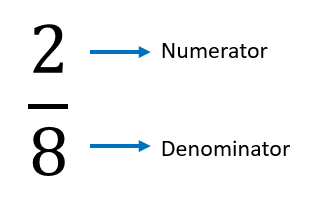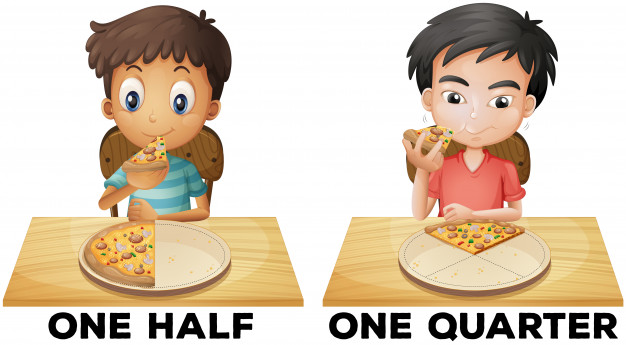Mathematics
Fractions and their Operations
9.7k views

 1. A Whole and its Parts 2. What are Fractions? 3. Types of Fractions 4. Mathematical Operations On Fractions 5. Addition of Fractions 6. Subtraction of Fractions 7. Multiplication of Fractions 8. Division Of Fractions 9. Summary

September 16, 2020

## A Whole and its Parts

Martin had two equal-sized chocolate bars with different placement of grooves such that he could divide one of them into four pieces and the other one into just two pieces. He gave one piece to each of his six friends. Each one kept wondering if they got an equal share. To answer such questions, fractions come into play! A fraction is a number representing a part of a whole or a group of objects. Let us understand fractions, in an interesting and easy manner, through this article.

## What are Fractions?

You have a part of something but not the complete thing.  If I ask you how much do you have, what will be your answer? Take for example the below scenario:

• You ate $$2$$ slices of pizza that has a total of $$8$$ slices
• You have $$1$$ piece from a cake that has a total of $$6$$ pieces

In both the above cases, you don’t have the complete object but only a part of it. In other words, you have a fraction of the object.

When any object is divided into equal numbers of parts and you pick up one or more of those parts, you have a fraction of the object.
Now one very important characteristic of these parts of a fraction is that:  Each part of a fraction has to be equal to other parts.

Next, let us understand how to represent a fraction. A fraction is represented by a combination of $$2$$ numbers arranged one above the other separated by a horizontal dash. The number above the separating line is called a Numerator and the number below is called a Denominator.The numerator represents the concerned portion (which may be the parts you have or the parts you don’t have depending on your concern or query) and the Denominator represents the total number of parts or the divisions of the whole or total number of objects in the group.
For example, if you ate $$2$$ slices out of $$4$$ equal slices, you can say that you ate \begin{align}\frac{2}{4}\end{align} of the pizza or half of the pizza. This means that you have $$2$$ equal parts out of $$4$$ equal parts. The $$4$$ equal parts together make up one object. So this means that you don’t have one complete object and neither do you have none of the objects. You have more than zero but less than one. That is you have a fraction of something.
Similarly, if you eat one more slice out of these, then you have eaten \begin{align}\frac{3}{4}\end{align} of the pizza and\begin{align}\frac{1}{4}\end{align} of the pizza is left or one quarter is left.

## Types Of Fractions:

There are $$3$$ types of fractions:
Depending on I eat a part of a pizza or a full pizza along with a part of another pizza, the fractions may be represented in different forms.

Proper fractions: When the Numerator is less than the Denominator, it is called as a Proper fraction
Eg. \begin{align}\frac{2}{5}, \frac{1}{3}\end{align}
Improper Fractions: When the Numerator is more than the Denominator, it is called as an Improper fraction
Eg. \begin{align}\frac{7}{5}, \frac{8}{3}\end{align}
Mixed fractions: A combination of a Whole number and a Proper fraction is called as a mixed fraction
Eg. \begin{align}2\frac{2}{5},\,\, 3\frac{2}{3}\end{align}

 \begin{align}\frac{2}{5}\end{align} \begin{align}\frac{6}{3}\end{align} \begin{align}5\frac{1}{4}\end{align} Proper Fraction Improper fraction Mixed Fraction

## Mathematical Operations On Fractions

Doing calculations on fractions is different than doing the same on the whole numbers. So let us try to understand the steps for performing the various mathematical operations on fractions in detail.

Before understanding how to add fractions, let us revise what numerator and denominator represent. The Denominator represents the total number of equal parts of an object. So suppose I ask you to add  \begin{align}\frac{2}{8}+\frac{3}{8}\end{align}  how will you do it?
Let us imagine a pizza with a total of $$8$$ slices. \begin{align}\frac{2}{8}\end{align} represents $$2$$ slices out of $$8$$ and \begin{align}\frac{3}{8}\end{align} represents $$3$$ slices out of $$8$$. So if you add these you will have $$5$$ slices out of $$8$$. Hence \begin{align}\frac{2}{8}+\frac{3}{8}=(\frac{2+3}{8})=\frac{5}{8}\end{align}
This is fairly simple. To add two or more fractions that have the same denominator, you will have to simply add the values in the numerator while keeping the denominator the same.

But what if the fractions have different denominators? This is a bit tricky but very simple if you understand the concept. To add two or more fractions that have different denominators, you will first need to make the denominator across all fractions the same by finding the Least Common Multiple (LCM) of the denominators.

Once you have found the LCM, for each fraction, multiply the denominator with the multiplier so that the denominator becomes the LCM. At the same time, to keep the value of fraction constant, you also need to convert them into equivalent fractions by multiplying the numerator of the fraction with the same multiplier.

You repeat this operation for all the fractions so that all the fractions have the same denominator and then you can add the fractions easily.
Let us understand with an example. Suppose we want to add \begin{align}\frac{2}{5}+\frac{3}{4}\end{align}. Since these fractions have different denominators, we cannot simply add the numerators.

First, we have to convert the fractions so that they have the same denominator. The first step to do this is finding the LCM of $$5$$ and $$4$$. The LCM of $$5$$ and $$4$$ is $$20$$. So we will have to convert the fraction so that the denominator of the fraction becomes $$20$$

And at the same time, we don’t want to disturb the value of the fraction so we will multiply the individual numerators also with the respective multipliers.
Solve: \begin{align}\frac{2}{5}+\frac{3}{4}\end{align}

\begin{align}&=\frac{2\times 4}{5\times 4}+\frac{3\times 5}{4\times 5}\\&=\frac{8}{20}+\frac{15}{20}\\&=\frac{23}{20}\end{align}

Hence \begin{align}(\frac{2}{5}+\frac{3}{4})=\frac{23}{20}\end{align}

### Subtracting Fractions

Now since the addition of fractions is quite clear, subtracting fractions is very similar.
If you want to subtract fractions with the same denominator simply subtract the numerator while keeping the denominator the same. Refer to the example below:
Solve: \begin{align}\frac{5}{7}-\frac{1}{7}\end{align}

\begin{align}&=\frac{(5-1)}{7}\\ &=\frac{4}{7}\end{align}
Hence \begin{align}\frac{5}{7}-\frac{1}{7}=\frac{4}{7}\end{align}
If you want to subtract the fractions with different denominators, follow the same steps that we did for adding the fractions, and in the last step, subtract the numerators. Refer to the example below:
Solve:\begin{align}\frac{4}{5}-\frac{1}{2}\end{align}
\begin{align}&= \frac{{(4 \times 2)}}{{(5 \times 2)}} - \frac{{(1 \times 5)}}{{(2 \times 5)}}\\ &= \frac{8}{{10}} - \frac{5}{{10}}\\& = \frac{{8 - 5}}{{10}}\\ &= \frac{3}{{10}}\end{align}
Hence \begin{align}\frac{4}{5}-\frac{1}{2}=\frac{3}{10}\end{align}

### Multiplying Fractions

Multiplying fractions is fairly simple. When multiplying two or more fractions, the numerator of the solution will be the product of numerators of all fractions and the denominator will be the multiplication result or product of denominators of all the fractions. Refer to the example below:
Solve:\begin{align}\frac{3}{4}\times\frac{4}{7}\end{align}
\begin{align}&= \frac{{3 \times 4}}{{5 \times 7}}\\ &= \frac{{12}}{{35}}\end{align}
Hence \begin{align}(\frac{3}{5}\times\frac{4}{7})=\frac{12}{35}\end{align}

### Dividing Fractions

The method to divide fractions follows the same steps as multiplying fractions but with only one additional step. This additional step is to convert the divide fraction operation into multiplication operation. This is done by exchanging the numerator and denominator of the second fraction in the divide operation.
Take for example the below example:

Solve: \begin{align}\frac{2}{5}\div\frac{5}{8}\end{align}
To solve this example, we will
Replace the numerator and denominator of the second fraction (\begin{align}\frac{5}{8}\end{align}will become\begin{align}\frac{8}{5}\end{align})
Replacing the division symbol with multiplication
Solving the equation as a multiplication operation
\begin{align}\frac{2}{5}\div\frac{5}{8}\end{align}
\begin{align} &= \frac{{2 \times 8}}{{5 \times 5}}\\ &= \frac{{16}}{{55}} \end{align}
Hence\begin{align}\frac{2}{5}\div\frac{5}{8}=\frac{16}{25}\end{align}## Summary

Fractions are a very interesting concept in mathematics and lay a solid foundation for advanced levels in mathematics. Fractions also have a wide range of real-world applications. Once you become well versed with fractions, you can solve many real-life examples from daily life.